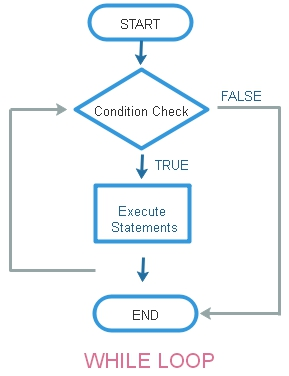# while loop in C programmingUsing while loop we will check the condition first and then execute the statement. Here the C statements can be executed only if condition is True or satisfied.

Usually the condition changes during the execution of the statement so next time while checking the condition we may get a False output. What will happen if the condition is never fails ( always satisfied ) ? We will read it here . Let us start with a basic program to print number 1 to 5.
``````#include<stdio.h>
int main()
{
int i=1;
while (i <=5){
printf("%d  \n",i); // Output
i=i+1; // increment of i by 1
}
return 0;
}``````
The above code will give this output.
``````1
2
3
4
5``````

## Indefinite loop

If we don’t increment the value of I inside the while loop, then the condition will be true in all cases and the loop will never end. This will continuously execute the code inside the loop without any end.
Avoid such loops as they never stops.
``````#include<stdio.h>
int main()
{
int i=1;
while (i <=5){
printf("%d  \n",i); // Output
}
return 0;
}``````
Sometime even the increment conditions became in such a way that the condition never became false and it became indefinite loop.
``````#include<stdio.h>
int main()
{
int i=1;
while (i <=5){
printf("%d  \n",i); // Output
i=i-1; // decrement of i by 1
}
return 0;
}``````

## Break statement

We can terminate the execution of loop in between and came out of the loop by using break statement.
Here we have used one if condition checking and once the value of i became more than 3 then break; statement gets executed and the loop is terminated.
``````#include<stdio.h>
int main()
{
int i=1;
while (i <=5){
printf("%d  \n",i); // Output
i=i+1; // increment of i by 1
if(i>3){
break;
}
}
return 0;
}``````
Output is here
``````1
2
3``````
We will increment the variable by more steps.
``````i++; // increment i value by 1
i--; // decrement i value by 1
i += 5; // increment i value by 5
i -= 5; // Decrement i value by 5 ``````

## Example with decrement of value by 10

``````#include<stdio.h>
int main()
{
int i=50;
while (i >= 0){
printf("%d  \n",i); // Output
i-=10; // increment of i by 1
}
return 0;
}``````
Output is here
``````50
40
30
20
10
0``````

## Reversing a string without using strlen()

strlen()
``````#include<stdio.h>
#include<string.h>
int main()
{
char str="plus2net";
int str_length;
str_length=strlen(str); // length of the string
while(str_length>=0){
printf("%c",str[str_length]);
str_length--;
}
return 0;
}``````